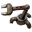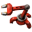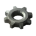## each * each = each

Post your ideas and suggestions how to improve the game.

Moderator: ickputzdirwech

kanvil
Burner InserterPosts: 7
Joined: Fri Jan 13, 2017 5:48 pm
Contact:

### each * each = each

##### TL;DR
Allow arithmetic combinators to have Each as both operands.
##### What ?
If both operands for an arithmetic combinators are set to Each, the output should be the result of the operand being applied to each input signal with itself.

i.e. If the input is { 5 plastic, 3 stone } and the operand is *, the output should be { 25 plastic, 9 stone }.
##### Why ?
This would give some interesting new capabilities to circuit networks. For example:

You could use a vector B where select signals = 1 to filter the specified signals from the vector A by computing:
output = ((A+B)^2 - A^2 - B^2) / 2

Nidan
Long Handed InserterPosts: 58
Joined: Sat Nov 21, 2015 1:40 am
Contact:

### Re: each * each = each

Arithmetic combinators can do exponentiation, so for the case of each*each you can use each^2 instead.

ssilk
Global ModeratorPosts: 12273
Joined: Tue Apr 16, 2013 10:35 pm
Contact:

### Re: each * each = each

Me also sees no other use case.
Cool suggestion: Eatable MOUSE-pointers.
Have you used the Advanced Search today?
Need help, question? FAQ - Wiki - Forum help
I still like small signatures...